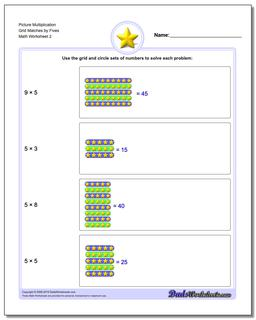# Math Worksheets: Picture Math Multiplication: Picture Math Multiplication: Picture Multiplication Grid Matches by Fives (Second Worksheet)## Picture Multiplication Grid Matches by Fives (Second Worksheet)

PropertyValue
DescriptionPicture Multiplication Grid Matches by Fives: Worksheets where students use visual grids to solve multiplication problems. These worksheets are a great first introduction to multiplication concepts for third grade students. (Second Worksheet)
Resource TypeWorksheet